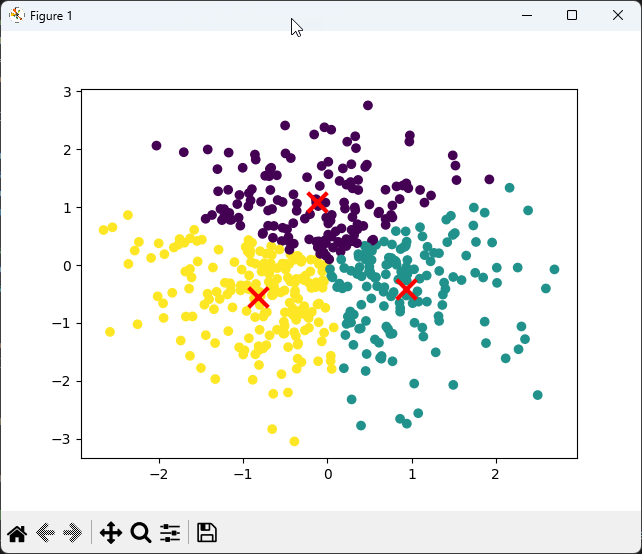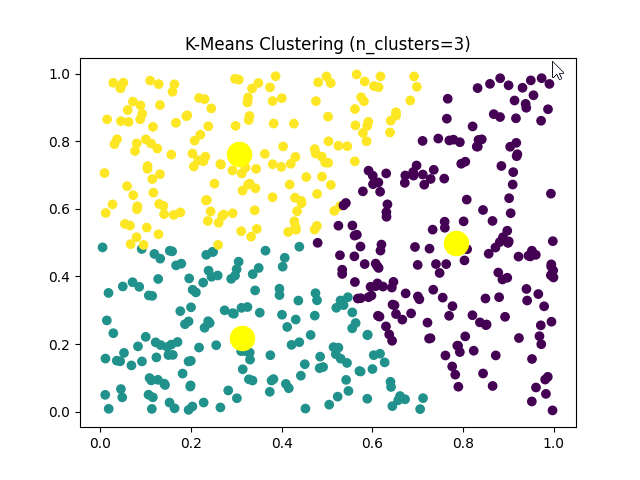Python

K Cluster

In this note, I want to try to apply an approach that is completely from other notes. I wanted to use chatGPT to create a Python code that I want instead of writing it myself.

NOTE : Refer to this note for my personal experience with chatGPT coding and advtantage & limitation of the tool. In general, I got very positive impression with chatGPT utilization for coding.

This code is created first by chatGPT on Feb 03 2023 (meaning using chatGPT 3.5) and then modified a little bit my me. The initial request that I put into chatGPT is as follows :

 Write a python script for the demo of K clustering method with following requirement.   1. Do not import any specific library specialized for Data analysis (only numpy and matplotlib are allowed if necessary) 2. Define following parameters (a ~ j) as mandatory a)n_data : the number of sample data which will be generated automatically by the program b)n_clusters: The number of clusters to form as well as the number of centroids to generate. c)init: The method for initialization of the centroids. The options are 'k-means++', 'random', or an ndarray. d)n_init: Number of time the k-means algorithm will be run with different centroid seeds. The final results will be the best output of n_init consecutive runs in terms of inertia. e)max_iter: Maximum number of iterations of the k-means algorithm for a single run. f)tol: Relative tolerance with regards to inertia to declare convergence. g)precompute_distances: Precompute distances (faster but takes more memory). h)verbose: Verbosity mode. i)random_state: Determines random number generation for centroid initialization. Pass an int for reproducible results. j)copy_x: When pre-computing distances it is more numerically accurate to center the data first. If copy_x is True, then the original data is not modified. Setting copy_x to False may be faster for large datasets. 3. Generate a set of arbitrary data (the size of the set is n_data) 4. Implement the K clustring algorithm for the given data 5. Plot the data set with each cluster in different colors

NOTE : It is not guaranteed that you would have the same code as I got since chatGPT produce the answers differently depending on the context. And it may produce the different answers everytime you ask even with the exact the same question.

NOTE : In this code, the requirements step 2) is also generated by chatGPT based on my question : "give me the list of parameters for K clustering.

NOTE : If you don't have any of your own idea for the request, copy my request and paste it into the chatGPT and put additional requests based on the output for the previous request. I would suggest to create a new thread in the chatGPT and put my request and then continue to add your own request.

 Kclustring_01.py import numpy as np import matplotlib.pyplot as plt import random   # NOTE : following description about the function is also generated by chatGPT based on my request. # This is the description of this function. # 1) Initialization: randomly initialize the centroids. The number of centroids is determined by the user and is equal to n_clusters. # 2) Assignment: assign each data point to the nearest centroid. This can be done by calculating the Euclidean distance between each data point and each centroid. # 3) Recomputation: calculate the mean of all the data points assigned to each centroid and use this mean as the new centroid. # 4) Repeat steps 2 and 3 until either the maximum number of iterations (max_iter) is reached or the difference between the old centroids and the new centroids is less than a user-defined tolerance (tol). # 5) Labeling: assign a label to each data point based on the centroid it was assigned to. # 6) Plotting: scatter plot the data points and color code them based on their labels. Plot the centroids as well, in a different color. # 7) Evaluation: Evaluate the performance of the K-Means algorithm by comparing the true labels of the data with the predicted labels obtained by the algorithm.   def kmeans(data, n_clusters, init, n_init, max_iter, tol, precompute_distances, verbose, random_state, copy_x):     n_samples, n_features = data.shape          # Randomly initialize the centroids     if init == 'k-means++':         centroids = np.zeros((n_clusters, n_features))         centroids = data[np.random.randint(n_samples), :]         for i in range(1, n_clusters):             dist = np.array([min([np.linalg.norm(data[j] - centroids[k]) for k in range(i)]) for j in range(n_samples)])             probs = dist/dist.sum()             cumprobs = probs.cumsum()             r = np.random.rand()             for j, p in enumerate(cumprobs):                 if r < p:                     break             centroids[i] = data[j, :]     elif init == 'random':         centroids = data[np.random.randint(n_samples, size=n_clusters), :]     else:         centroids = init              # Perform the K-means algorithm     for n in range(n_init):         centroids_old = centroids.copy()         # Assign the samples to the nearest centroids         labels = np.array([np.argmin([np.linalg.norm(data[i]-centroids[j]) for j in range(n_clusters)]) for i in range(n_samples)])         # Compute the new centroids as the mean of the samples assigned to each centroid         for i in range(n_clusters):             centroids[i] = np.mean(data[labels == i], axis=0)         # Check if the centroids have changed         if np.linalg.norm(centroids - centroids_old) < tol:             break     # Plot the results     plt.scatter(data[:, 0], data[:, 1], c=labels)     plt.scatter(centroids[:, 0], centroids[:, 1], marker='x', s=200, linewidths=3, color='r')     plt.show()     # Define the parameters n_data = 500 n_clusters = 3 init = 'k-means++' n_init = 10 max_iter = 300 tol = 1e-4 precompute_distances = False verbose = False random_state = None copy_x = True   # Generate arbitrary data np.random.seed(0) data = np.random.randn(n_data, 2)     # Perform the K-means clustering kmeans(data, n_clusters, init, n_init, max_iter, tol, precompute_distances, verbose, random_state, copy_x)

The result from this code is as follows :As a next step, I asked chatGPT to simplify the code using a any package that can simplify the same implementation and the test routine. The requested code and the result are as follow.

 Kclustring_03.py import numpy as np import matplotlib.pyplot as plt import random import numpy as np from sklearn.cluster import KMeans import matplotlib.pyplot as plt   def kmeans(data, n_clusters, n_init=10, max_iter=300, tol=0.0001, random_state=0):     kmeans = KMeans(n_clusters=n_clusters, n_init=n_init, max_iter=max_iter, tol=tol, random_state=random_state)     kmeans.fit(data)     labels = kmeans.labels_     centroids = kmeans.cluster_centers_     return labels, centroids   def display_result(X, y_pred, n_clusters, kmeans):     plt.scatter(X[:, 0], X[:, 1], c=y_pred)     plt.scatter(kmeans.cluster_centers_[:, 0], kmeans.cluster_centers_[:, 1], s = 300, c = 'yellow', label = 'Centroids')     plt.title(f'K-Means Clustering (n_clusters={n_clusters})')     plt.show()   def test_kmeans():     # Generate random sample data with 100 samples and 2 features     n_samples = 500     n_features = 2     X = np.random.rand(n_samples, n_features)     n_clusters = 2          # Call the kmeans function     labels, centroids = kmeans(X, n_clusters)          # Assert that the shape of the labels is correct     assert labels.shape == (n_samples,), f"Expected shape {(n_samples,)}, but got {labels.shape}"          # Assert that the shape of the centroids is correct     assert centroids.shape == (n_clusters, n_features), f"Expected shape {(n_clusters, n_features)}, but got {centroids.shape}"          # Create an instance of the KMeans class     kmeans_model = KMeans(n_clusters=n_clusters, n_init=10, max_iter=300, tol=0.0001, random_state=0)     kmeans_model.fit(X)          # Display the result     display_result(X, labels, n_clusters, kmeans_model)   test_kmeans()

The result of the code is as shown below.